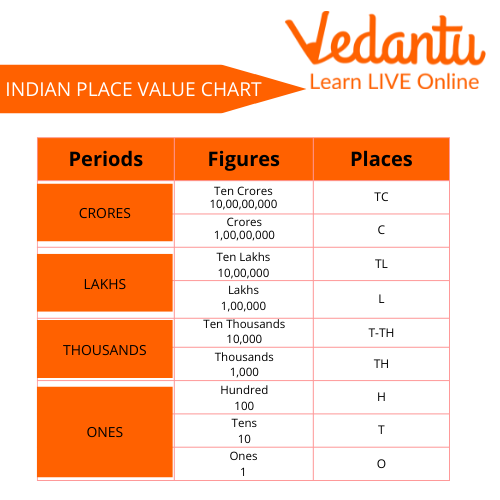Courses
Courses for Kids
Free study material
Free LIVE classes
More

# Let’s Write the Place Value of NumbersLIVE
Join Vedantu’s FREE Mastercalss

## What is the Place Value?

A place value of the digit is the value decided by the position of the digit. There is a value of every digit decided by its place. The place value of the same digits in a number can be different. The place value of the digits is decided from right to left. The value starts deciding from the right, called the one's position.

In a number, the place value of digits is ones, tens, hundred, thousand, ten thousand, and so on. These values are decided by the position and differ. No two digits can have the same value in the number. We will see how to find the place value and how to write the place value of a digit.

## How to Find the Place Value?

The place value of a digit is decided by the position of a digit in the number. As the value of two digits cannot be the same in a single number. Let us understand how the place value of a digit is decided.Place Value

Through this example, we can see that the digit at the right, which is 8, is given the place value of ones/units. The digit left to one is 6, given the place value of Tens. The digit left to the tens is 4 given the place value of hundred, and so on.

To find out the place value in number format, we multiply the number with its place value. As in this example, the digit at the rightmost place is multiplied by 1, the digit at the tens place is multiplied by 10, the digit at the hundredth place is multiplied by 100, and so on.

## Indian Place Value Chart

There is an Indian Place Value Chart and International Place Value Chart. In the Indian Place Value Chart, the digits are determined as ones, tens, hundred, thousand, ten thousand, lakhs, ten lakhs, crore, and ten crores.

These values are determined by the position of a digit in a number. The place value of any two same digits cannot be the same. The place value of each digit in a number is different.Indian Place Value Chart

## Place Value and Face Value

A face value of a digit is the digit itself. The face value of a digit is determined by the mirror value of the digit, while the place value is determined by the position of the digit. Let us take an example to understand the differentiation between the two.

• The number is 5678.

• The place value of 8 is 8, 7 is 70, 6 is 600, and 5 is 5000.

• The face value of 8 is 8, 7 is 7, 6 is 6, and 5 is 5.

Hence, we can say that the face value of the digit is the digit itself, and the place value is decided by the position of the digit in a number.

## Solved Questions

Q1. Write the place value of 2 in 324.

Ans: To find out the place value of 2 in 324, we have to see if 2 is at one, tens, or hundredth place. Here 2 is the tenth place, so we have to multiply 2 by 10. The place value of 2 is 20.

Q2.  Write the place value of 7 in 27.

Ans: To find out the place value of 7 in 27, we must see if 7 is at one or tenth place. Here 7 is at one place, so we have to multiply 7 by 1. The place value of 7 is 7.

## Practice Questions

Q1. Write the place value of 8 in 8756.

Ans: 8000.

Q2. Write the place value of 6 in 68.

Ans: 60.

## Summary

Finding a place value of a digit is an important concept to learn in Mathematics. It is required in the chapters of addition, subtraction, division, and multiplication as well. This concept teaches the kids to place the digits correctly in a number.

The place value of a digit and the face value of a digit is also different. There is an Indian Place Value Chart and International Place Value Chart. In India, we follow the Indian Place Value Chart in which the values are decided as Ones, Tens, Hundred, Thousands, Ten Thousand, Lakhs, Ten Lakhs, and so on.

Last updated date: 24th Sep 2023
Total views: 113.7k
Views today: 3.13k

## FAQs on Let’s Write the Place Value of Numbers

1. Why is the place value of numbers important?

The concept of place value in numbers is important because it gives the worth of every digit in a number according to its position. The essence of numbers as a whole can only be understood through the knowledge of place values.

2. What is the difference between the place value and the face value of a digit? Explain the same with an example.

The place value of a digit in a number is decided by its position in a number. The place value of every digit in a number is different. On the other hand, the face value of a number is the number itself. The face value of every digit in a number is different too.

3. Explain the concept of face value. Write the face value of 2 in 7624.

The face value of any digit in the number is the same digit. We always write the same digit as the face value of a digit. Let us see an example to understand this. In 3562 the face value of 3 would be 3, 5 would be 5, 6 would be 6, and 2 would be 2. In the same way, the face value of 2 in 7624 would be 2 itself. This concept is followed in the face-value questions.